# Go Math Grade 3 Chapter 2 Answer Key Pdf Represent and Interpret Data

Go Math Grade 3 Chapter 2 Answer Key Pdf: Students who are searching for Go Math Grade 3 Answer Key Chapter 2 Represent and Interpret Data pdf can get them here. Go Math Grade 3 Solution Key Chapter 2 Represent and Interpret Data includes all the important lessons which helps to improve your math skills. If you want to get the highest marks in the exam then you must practice HMH Go Math Grade 3 Answer Key Chapter 2 Represent and Interpret Data. We have covered all the topics in this chapter with brief explanation.

## Represent and Interpret Data Go Math Grade 3 Chapter 2 Answer Key Pdf

Before you start solving the problems we request you go through all the topics covered in this chapter. Students who want to become a master in math must work hard from the primary level itself. So, Download Free Pdf Go Math Grade 3 Answer Key Represent and Interpret Data. This helps you to improve your skills in math and become and champ in that subject. Test your knowledge by solving the problems Grade 3 Go Math Answer Chapter Represent and Interpret Data Review/Test. The topics covered in this chapter are given below.

Lesson 1: Problem Solving • Organize Data

Lesson 2: Use Picture Graphs

Lesson 3: Make Picture Graphs

Mid-Chapter Checkpoint

Lesson 4: Use Bar Graphs

Lesson 5: Make Bar Graphs

Lesson 6: Solve Problems Using Data

Lesson 7: Use and Make Line Plots

Chapter 2 Review/Test

### Organize Data Page No 91

Problem Solving Organize Data

Use the Favorite School Subject tables for 1–4.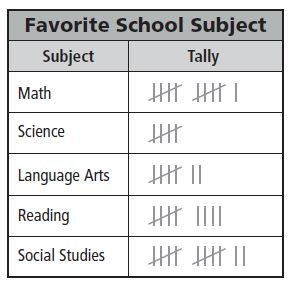Question 1.
The students in two third-grade classes recorded their favorite school subject. The data are in the tally table. How many fewer students chose science than chose social studies as their favorite school subject?
Think: Use the data in the tally table to record the data in the frequency table. Then solve the problem.
social studies: 12 students
science: 5 students
12 – 5 = 7
So, 7 fewer students chose science.

 Subject Number Math ____________ Science 5 Language Arts ____________ Reading ____________ Social Studies 12

 Subject Number Math 11 Science 5 Language Arts 7 Reading 9 Social Studies 12

Question 2.
What subject did the least number of students choose?
___________

Explanation:

We can answer the question by using the above tally table. The table shows the least number of students is 5. Thus the answer is Science.

Question 3.
How many more students chose math than language arts as their favorite subject?
_______ more students

Explanation:

If we look at the above table, there are 11 students who chose Math and 7 students who chose the language arts

To know the students who chose math than language arts we have to subtract 11 and 7
= 11 – 7
= 4
Thus the students chose math than language arts as their favorite subject are 4

Question 4.
Suppose 3 students changed their vote from math to science. Describe how the frequency table would change.

Type below:
__________

Answer: There would be an equal number of students who chose math and who chose science

Explanation:

If we look at the graph there are 11 students who voted for Math and 5 students who voted for Science
If 3 students changed their vote from math to science then the new graph will be

11- 3= 8
i.e., Actual No. of Science Students + New Students who changed from Math to Science
= 5 + 3
= 8

### Organize Data Lesson Check Page No 92

Question 1.
The tally table shows the cards in Kyle’s sports card collection.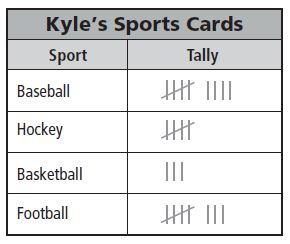How many hockey and football cards does Kyle have combined?
Options:
a. 5
b. 8
c. 12
d. 13

Explanation:

Given,
Kyle has 5 hockey cards and 8 football cards
To know total no. of hockey and football cards does Kyle have combined
We have to add 5 + 8 = 13
Therefore the total no. of cards that Kyle have combined is 13

Spiral Review

Question 2.
There are 472 people in the concert hall. What is 472 rounded to the nearest hundred?
Options:
a. 400
b. 470
c. 500
d. 600

If the digit to the right is more or greater than 5, then the digit in the rounding place will be increased to 1.
472 is greater than 450
So, 472 rounded to the nearest hundred is 500
So the answer is option c.

Question 3.
Max and Anna played a video game as a team. Max scored 463 points and Anna scored 329 points. How many points did they score in all?
Options:
a. 892
b. 792
c. 782
d. 134

Explanation:

Given that,
Max scored 463 points and,
Anna scored 329 points
To know the total points they scored
We need to add both Max and Anna points
i.e., 436 + 329 = 792

Question 4.
Judy has 573 baseball cards in her collection. Todd has 489 baseball cards in his collection. How many fewer cards does Todd have than Judy?
Options:
a. 84
b. 94
c. 116
d. 184

Explanation:

Given,
Judy has 573 baseball cards in her collection
Todd has 489 baseball cards in his collection
To find how many fewer cards does Todd have than Judy
We have to find the difference between Judy and Todd baseball cards
= 573 – 489 = 84

Question 5.
Ms. Westin drove 542 miles last week and 378 miles this week on business. How many miles in all did she drive on business during the two weeks?
Options:
a. 810 miles
b. 820 miles
c. 910 miles
d. 920 miles

Explanation:

We observe that Ms. Westin drove 542 miles last week and 378 miles this week on business
Total number of miles in all did she drive on business during the two weeks is?
542 + 378 = 920 miles
Thus the answer to the above question is option d.

### Use Picture Graphs Page No 97

Use the Math Test Scores picture graph for 1–7.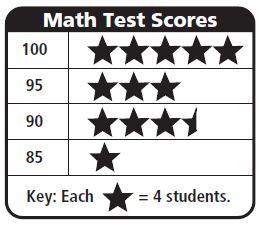Question 1.
How many students scored 100? How can you find the answer?

Answer: To find the number of students who scored 100, count each star as 4 students. So, 20 students scored 100.

Question 2.
What does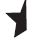stand for?
________ students

Explanation:

The full star stands for 4 students
That means the half star is equal to two stars.

Question 3.
How many students in all scored 100 or 95?
________ students

Explanation:

No. of students who scored 100 = 5 stars
Each star = 4 students
i.e., 5 × 4 = 20 students
No. of students who scored 95 = 3
Each star = 4 students
That means 3 × 4 = 12
Total No. of students in all scored 100 or 95
12 + 20 = 32
Thus the answer is 32 students

Question 4.
How many more students scored 90 than 85?
________ students

Explanation:

Students who scored 90 = 3 and a half star = 4 + 4 + 4 + 2
Students who score 85 = 1 star = 4
That means students scored 90 than 85 are
14 – 4 = 10 students

Question 5.
How many students in all took the test?
________ students

Explanation:

Students who scored 100 (5 stars) = 4 + 4 + 4 + 4 + 4 = 20 student
Students who scored 95 (3 stars) = 4 + 4 + 4 = 12 students
Students who scored 90 (3 and a half star) = 4 + 4 + 4 + 2 = 14 students
Students who score 85 (1 star) = 4 students
Total No. of students who took test = 20 + 12 + 14 + 4 = 50 students

Problem Solving

Question 6.
Suppose the students who scored 85 and 90 on the math test take the test again and score 95. How many stars would you have to add to the picture graph next to 95?
Type below:
__________

Answer: 4 Stars and half of a star

Explanation:

Students who scored 90 = 3 and a half star
Students who score 85 = 1 star
That means students scored 90 than 85 = 4 and a half star
Thus 4 and a half star stars would you have to add to the picture graph next to 95

Question 7.
If 2 more students took the math test and both made a score of 80, what would the picture graph look like?
Type below:
__________

Answer: There would be another row below 85. There would be half of a star next to 80.

Explanation:

There would be 5 lines and the 5th line will contain a half star

### Use Picture Graphs Lesson Check Page No 98

Question 1.
Karen asked her friends to name their favorite type of dog.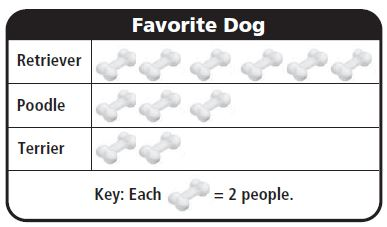How many people chose poodles?
Options:
a. 10
b. 6
c. 4
d. 3

Explanation:

If we look at the graph, there are three bones for poodles.
Each bone represents 2 people, which means 3 bones represent 6 people.
2 + 2 + 2 = 6 people chose poodles

Question 2.
Henry made a picture graph to show what topping people like on their pizza. This is his key.
Each= 6 people.
What does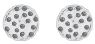stand for?
Options:
a. 2 people
b. 6 people
c. 9 people
d. 12 people

Explanation:

By seeing the picture graph we can say that
Each pizza = 6 people
Then 2 pizzas = 6 + 6
= 12 people
S, the correct answer is option D

Spiral Review

Question 3.
Estimate the sum.
5 2 3
+ 2 9 5
———
Options:
a. 900
b. 800
c. 700
d. 600

Explanation:

Sum of 523 and 295
= 818
As 818 is 850 the estimated sum of 523 and 295 is 800

Thus the correct answer is 800

Question 4.
Estimate the difference.
6 1 0
– 1 8 7
——-
Options:
a. 800
b. 600
c. 500
d. 400

Explanation:

Subtract 610 and 187
We get 413
413 is less than 450 and is nearer to 400
So, the estimated difference of 610 and 187 is 400

Question 5.
What is 871 rounded to the nearest ten?
Options:
a. 900
b. 880
c. 870
d. 800

Explanation:

If the digit is less than 5 then the number will be decreased by 1
So, the number 871 rounded to the nearest ten is 870

Question 6.
What is 473 rounded to the nearest hundred?
Options:
a. 400
b. 470
c. 500
d. 570

Explanation:

473 is greater than 450 so it must be increased
473 rounded to the nearest hundred is 500
So, the correct answer is an option (C)

### Make Picture Graphs Page No 103

Ben asked his classmates about their favorite kind of TV show. He recorded their responses in a frequency table. Use the data in the table to make a picture graph.Follow the steps to make a picture graph.
Step 1 Write the title at the top of the graph.
Step 2 Look at the numbers in the table. Tell how many students each picture represents for the key.
Step 3 Draw the correct number of pictures for each type of show.
Use your picture graph for 1–5.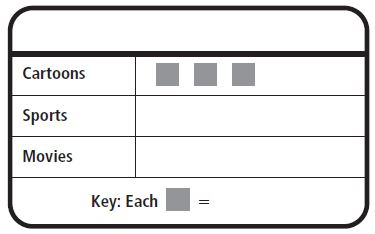Question 1.
What title did you give the graph?
Type below:
_________

Question 2.
What key did you use?
________

Answer: Each ■ = 3 students

Question 3.
How many pictures did you use to represent sports?
_______ pictures

Problem Solving

Question 4.
How many pictures would you draw if 12 students chose game shows as their favorite kind of TV show?
________ pictures

Question 5.
What key would you use if 10 students chose cartoons?
■ = ______ students

Explanation:

If 10 student chose cartoons, we can use a key that is a factor of 10
■■■■■ = 10
and each ■ = 2 students

### Make Picture Graphs Lesson Check Page No 104

Question 1.
Sandy made a picture graph to show the sports her classmates like o play. How many fewer students chose baseball than chose soccer?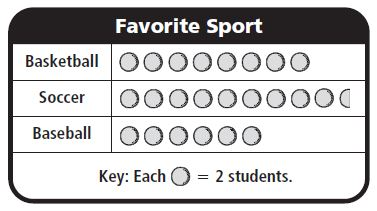Options:
a. 3
b. 4
c. 7
d. 8

Explanation:

Students chose Soccer = 9 and a half ball
Students chose Baseball = 6 balls
Given each ball = 2 students
So, students chose soccer = 2+2+2+2+2+2+2+2+2+1
=19 students
Students chose baseball = 2+2+2+2+2+2
= 12 students
students chose baseball than chose soccer = 19 – 12
= 7 students

Question 2.
Tommy is making a picture graph to show his friends’ favorite kind of music. He plans to use one musical note to represent 2 people. How many notes will he use to represent that 4 people chose country music?
Options:
a. 2
b. 4
c. 6
d. 8

Explanation:

Given, Tommy is making a picture graph to show his friends’ favorite kind of music
One musical note = 2 people
For 4 people =?
2 + 2 people = 2 musical notes

Spiral Review

Question 3.
Find the sum.
4 9 0
+ 2 3 4
———
Options:
a. 256
b. 624
c. 664
d. 724

Addition of 490 and 234 = 724

Question 4.
Sophie wrote odd numbers on her paper. Which number was NOT a number that Sophie wrote?
Options:
a. 5
b. 11
c. 13
d. 20

Explanation:

Examples of odd numbers are 1,3,5,7,9,11,13,15….
20 is an even number
So, the number was NOT a number that Sophie wrote is 20
Thus the correct answer is 20

Question 5.
Miles ordered 126 books to give away at the store opening. What is 126 rounded to the nearest hundred?
Options:
a. 230
b. 200
c. 130
d. 100

Explanation:

126 here 1 is rounded which is in hundred place
If the number is greater than 150 then it would equal to 200
But it is less than 150, so, 126 rounded to the nearest hundred is 100
Thus the correct option is D

Question 6.
Estimate the difference.
4 2 2
– 2 8 4
——–
Options:
a. 100
b. 180
c. 200
d. 700

Explanation:

The subtraction of 422 and 284 is 138
138 is less than 150, so the estimated difference of 422 and 284 is 100.
Thus the correct answer is option (A)

### Mid-Chapter Checkpoint Page No 105

Vocabulary

Choose the best term from the box.Question 1.
A __________ uses numbers to record data.
_________

Question 2.
A __________ uses small pictures or symbols to show and compare information.
_________

Concepts and Skills

Use the Favorite Season table for 3-6.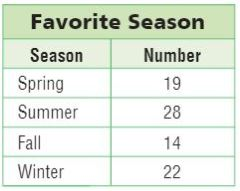Question 3.
Which season got the most votes?
_________

From the above table, we can say that the highest number of votes is for Summer i.e., 28

Question 4.
Which season got 3 fewer votes than winter?
_________

Explanation:

Number of votes for Winter = 22
Number of votes for Spring = 19
22 – 19 = 3
So, Spring season got 3 fewer votes than winter

Question 5.
How many more students chose summer than fall?
________ students

Explanation:

Number of students chose summer = 28
Number students chose fall = 14
To know the students chose summer than fall
We have to subtract votes for summer and fall
28 – 14 = 14
Therefore 14 more students chose summer than fall

Question 6.
How many students chose a favorite season?
________ students

Explanation:

Number of students chose summer =  28
Number of students chose winter = 22
Number of students chose spring = 19
Number of students chose fall = 14
Total Number of students chose favorite season = 28+22+19+14 = 83
The correct answer is 83 students

Use the Our Pets picture graph for 7-9.Question 7.
How many students have cats as pets?
________ students

Explanation:

Number of paws for cats = 5
Each paw = 2 students
2+2+2+2+2 = 10 students

Question 8.
Five more students have dogs than which other pet?
__________

Explanation:

Number of paws for dogs = 6 and a half paw
Each paw = 2 students
2+2+2+2+2+2+1 = 13 students
Number of paws for bird = 4
2+2+2+2 = 8 students
13 – 8 = 5 students

Question 9.
How many pets in all do students have?
_________ students

Explanation:

Number of paws for dogs = 6 and a half paw = 2+2+2+2+2+2+1 = 13 students
Number of paws for bird = 4 = 2+2+2+2 = 8 students
Number of paws for cats = 5 = 2+2+2+2+2 = 10 students
Number of paws for fish = 3 = 2+2+2 = 6 students
Total pets in all do students have = 13+8+10+6
= 37 students

### Mid-Chapter Checkpoint Lesson Check Page No 106

Use the Favorite Summer Activity picture graph for 10-14.Question 10.
Some students in Brooke’s school chose their favorite summer activity. The results are in the picture graph at the right. How many students chose camping?
________ students

Explanation:

Total students chose camping = 5
Each= 10 students
10+10+10+10+10 = 50

Question 11.
How many more students chose swimming than canoeing?
_______ students

Explanation:

Total students chose swimming = 6Each= 10 students
= 10+10+10+10+10+10 = 60 students
Total students chose canoeing = 3= 10+10+10 = 30 students
Total students chose swimming than canoeing = 60 – 30
= 30 students

Question 12.
Which activity did 15 fewer students choose than camping?
__________

Explanation:

Total students chose camping = 5
10+10+10+10+10 = 50 students
Total students chose biking = 3 and a half picture
10+10+10+5 = 35
Biking is the activity did 15 fewer students choose than camping

Question 13.
How many pictures would you draw for biking if each= 5 students?
_______

Explanation:

You would draw 7 pictures
If you look at the graph there are 35 students who chose biking
So, to represent 35 students when each picture represents 5 students, we will need 7 pictures
i.e., 5+5+5+5+5+5+5 = 35 students

Question 14.
How many more students choose swimming and camping combined than biking and canoeing?
_________ students

Explanation:

First of all, we need to find how many students chose swimming and camping combined
Total students chose swimming = 6Each= 10 students
= 10+10+10+10+10+10 = 60 students
Total students chose camping = 5
10+10+10+10+10 = 50 students
60+50 = 110 students
Next, we need to find how many students chose biking and canoeing
Total students chose canoeing = 3= 10+10+10 = 30 students
Total students chose biking = 3 and a half picture
10+10+10+5 = 35
30+35 = 65 students
Then, we need to subtract
110 – 65 = 45 students
Therefore the students choose swimming and camping combined than biking and canoeing = 45 students

### Use Bar Graphs Page No 111

Use the After-Dinner Activities bar graph for 1–6.

The third-grade students at Case Elementary School were asked what they spent the most time doing last week after dinner. The results are shown in the bar graph at the right.Question 1.
How many students spent the most time watching TV after dinner?
3 students

Explanation:

From the above bar graph, we can see the activities of the students after dinner
Students spent the most time watching TV after dinner is between 2 and 4 i.e., 3 students

Question 2.
How many students in all answered the survey?
_________ students

Explanation:

Total students who spent the most time reading after dinner = 6
Students who spent the most time doing homework after dinner = 12
Students who spent the most time watching TV after dinner = 3
Students who spent the most time playing a game after dinner = 8
Total students in all answered the survey = 6 + 12 + 3 + 8
= 29 students

Question 3.
How many students in all played a game or read?
__________ students

Explanation:

Students who spent the most time reading after dinner = 6
Students who spent the most time playing a game after dinner = 8
Total students in all played a game or read = 6 + 8
= 14 students

Question 4.
How many fewer students read than did homework?
__________ students

Explanation:

Students who spent the most time reading after dinner = 6
Students who spent the most time doing homework after dinner = 12
To find the students read than did homework = 12 – 6
= 6 students

Question 5.
How many more students read than watched TV?
________ students

Explanation:

Students who spent the most time reading after dinner = 6
Students who spent the most time watching TV after dinner = 3
To find the students read than watched TV = 6 – 3
= 3 students

Problem Solving

Question 6.
It would end at _________

Answer: Halfway between 8 and 10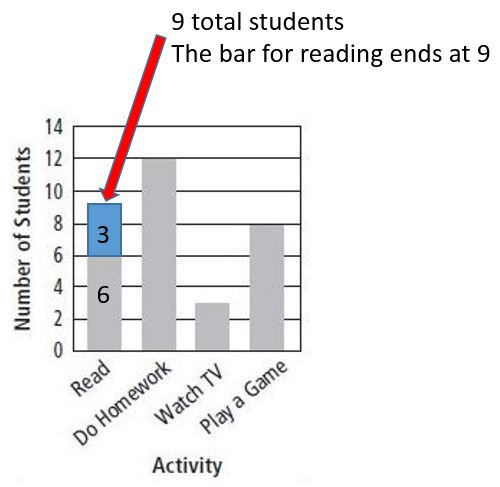Explanation:

According to the graph, Students who spent the most time reading after dinner = 6
If 3 more students changed their answers to reading instead of doing homework, the total students would be 9 i.e., 6 + 3

### Use Bar Graphs Lesson Check Page No 112

Question 1.The bar graph shows the number of sandwiches sold at Lisa’s sandwich cart yesterday. How many tuna sandwiches were sold?
Options:
a. 12
b. 16
c. 18
d. 20

Explanation:

According to the bar graph, tuna sandwiches sold at Lisa’s sandwich cart are between 16 to 20
The no. of tuna sandwiches were sold at Lisa’s sandwich cart = 18
So, the correct answer is option (C)

Spiral Review

Question 2.
What is 582 rounded to the nearest ten?
Options:
a. 500
b. 580
c. 590
d. 600

Explanation:

If the digit is less than 5 then the digit will be increased by 1.
582, 8 is rounded here.
2 < 5 so 582 rounded to the nearest ten is 580

Question 3.
Savannah read 178 minutes last week. What is 178 rounded to the nearest hundred?
Options:
a. 400
b. 280
c. 200
d. 180

Explanation:

Savannah read 178 minutes last week
178 is greater than 150, so the number 178 rounded to the nearest hundred is 200

Question 4.
Estimate the difference.
3 7 1
– 9 9
——-
Options:
a. 500
b. 400
c. 300
d. 200

Explanation:

The difference between 371 and 99 is 272
272 is near to 300. Because 272 is greater than 250.
So, the estimated difference between 371 and 99 is 300

Question 5.
Estimate the difference.
6 2 5
– 2 4 8
———
Options:
a. 800
b. 500
c. 400
d. 300

Explanation:

The difference between 625 and 248 is 377
377 rounded to the nearest hundred is 400
Therefore the estimated difference between 625 and 248 is 400.

### Make Bar Graphs Page No 117

Ben asked some friends to name their favorite breakfast food. He recorded their choices in the frequency table at the right.Question 1.
Complete the bar graph by using Ben’s data.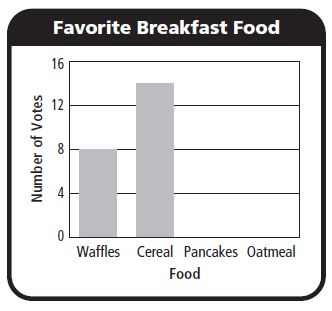Oatmeal = 4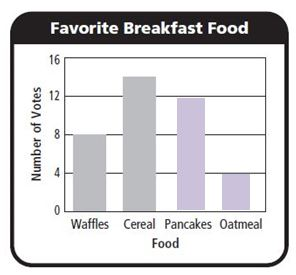Use your bar graph for 2–5.

Question 2.
Which food did the most people choose as their favorite breakfast food?
__________

Explanation:

From the above bar graph, we can say that most of the people chose Cereals as their favorite food.
Number of votes for cereals = 14

Question 3.
How many people chose waffles as their favorite breakfast food?
_________ people

Explanation:

The bar graph shows that the number of people who chose Waffles as their favorite breakfast food is 8.

Question 4.
How did you know how high to draw the bar for pancakes?
Type below:
__________

Since 12 people chose pancakes, I made the top of the bar end at the line for 12

Question 5.
Suppose 6 people chose oatmeal as their favorite breakfast food. How would you change the bar graph?
Type below:
___________

Answer: I would make the bar for oatmeal end halfway between 4 and 8.### Make Bar Graphs Lesson Check Page No 118Question 1.
Gary asked his friends to name their favorite pizza topping. He recorded the results in a bar graph. How many people chose pepperoni?
Options:
a. 6
b. 5
c. 4
d. 1

Explanation:

The bar graph shows that the number of people who chose pepperoni is 6
So, the correct answer is option (a)

Question 2.
Suppose 3 more friends chose mushrooms. Where would the bar for mushrooms end?
Options:
a. 2
b. 4
c. 6
d. 8

Explanation:

We notice that the vertical bar for mushrooms ends at 1
1 person chose mushrooms
If 3 more friends chose mushrooms, the bar would end at 4
Then the answer is 1 + 3 = 4

Spiral Review

Question 3.
Estimate the sum.
4 5 8
+ 2 1 4
———
Options:
a. 700
b. 600
c. 300
d. 200

Explanation:

Round 458 up to 500
Round 214 down to 200
Now, the estimated sum will be 500 + 200 = 700
So, the correct answer is option A

Question 4.
Matt added 14 + 0. What is the correct sum?
Options:
a. 140
b. 14
c. 1
d. 0

Explanation:

Any number added by 0 is itself. So the sum of 14 + 0 = 14
The correct answer is Option B

Question 5.
There are 682 runners registered for an upcoming race. What is 682 rounded to the nearest hundred?
Options:
a. 600
b. 680
c. 700
d. 780

Explanation:

If the digit to the right is more or equal than 5, then the digit in the rounding place increases by one
Change all the digits to the right of the rounding place to zero.
So, 682 rounded to the nearest hundred 700

The correct answer is option C

Question 6.
There are 187 new students this year at Maple Elementary. What is 187 rounded to the nearest ten?
Options:
a. 100
b. 180
c. 190
d. 200

Explanation:

If the digit to the right is more or equal than 5, then the digit in the rounding place increases by one
Change all the digits to the right of the rounding place to zero.
So, the number 187 rounded to the nearest ten is 190
Thus the correct answer is Option C

### Solve Problems Using Data Page No 123

Use the Favorite Hot Lunch bar graph for 1–3.Question 1.
How many more students chose pizza than chose grilled cheese?
Think: Subtract the number of students who chose grilled cheese, 2, from the number of students who chose pizza, 11.
11 – 2 = 9

Question 2.
How many students did not choose chicken patty?
__________ students

Explanation:

Number of students who chose hot dog = 8
Number of students who chose Pizza = 11
Number of students who chose grilled cheese = 2
Number of students who chose Chicken Patty = 5
Total Number of students who did not choose the chicken patty = 8 + 11 + 2 = 21

Question 3.
How many fewer students chose grilled cheese than chose hot dogs?
__________ fewer students

Explanation:

Number of students who chose hot dog = 8
Number of students who chose grilled cheese = 2
Subtract the number of students who chose grilled cheese from the number of students who chose a hot dog
= 8 – 2 = 6
Therefore, 6 fewer students chose grilled cheese than chose hot dogs

Use the Ways to Get to School bar graph for 4–7.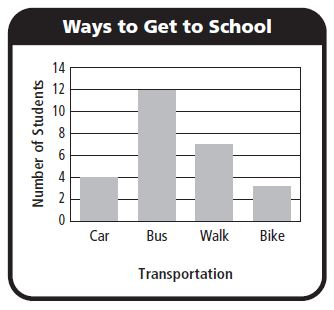Question 4.
How many more students walk than ride in a car to get to school?
________ more students

Explanation:

No. of students walk to get to school = 7
No. of students ride in a car to get to school = 4
Now, subtract the no. of students walk from no. of students ride in a car
We get, 7 – 4 = 3

Question 5.
How many students walk and ride a bike combined?
________ students

Explanation:

Number of students walk to get to school = 7
Number of students ride a bike to get to school = 3
To know how many students walk and ride a bike combined
We have to add Number of students walk and ride a bike
= 7 + 3 = 10

Problem Solving

Question 6.
Is the number of students who get to school by car and bus greater than or less than the number of students who get to school by walking and biking? Explain.
Options:
a. greater
b. less

Explanation:

4 + 12 = 16; 7 + 3 = 10; 16 > 10.

Question 7.
What if 5 more students respond that they get to school by biking? Would more students walk or ride a bike to school? Explain.
________

Explanation:

7 students walk; 3 + 5 = 8 students bike

7 < 8

### Solve Problems Using Data Lesson Check Page No 124Question 1.
How many fewer votes were for bench repair than for food drive?
Options:
a. 9
b. 10
c. 16
d. 11

Explanation:

Number of votes for food drive = 13
Number of votes for bench repair = 3
To find votes were for bench repair than for food drive
We need to subtract Number of votes for bench repair from Number of votes for food drive
i.e., 13 – 3 = 10

Question 2.
How many votes were there in all?
Options:
a. 14
b. 4
c. 32
d. 34

Explanation:

Number of votes for food drive = 13
Number of votes for bench repair = 3
Number of votes for Wall Mural = 10
Number of votes for Park Pick up = 6
Total no. of votes = 13 + 10 + 3 + 6 = 32

Spiral Review

Question 3.
Find the difference.
6 5 0
– 1 8 9
——–
Options:
a. 461
b. 479
c. 539
d. 571

Explanation:

Here we have to subtract 650 from 189
650 – 189 = 461

Question 4.
Greyson has 75 basketball cards. What is 75 rounded to the nearest ten?
Options:
a. 60
b. 70
c. 80
d. 90

Explanation:

If the digit to the right is more or equal than 5, then the digit in the rounding place increases by one
Change all the digits to the right of the rounding place to zero.
So, 75 rounded to the nearest ten is 80

Question 5.
Sue spent $18 on a shirt,$39 on a jacket, and $12 on a hat. How much did she spend in all? Options: a.$79
b. $69 c.$57
d. $51 Answer:$69

Explanation:

Given
Sue spent $18 on a shirt Sue spent$39 on a jacket and $12 on a hat Total amount she spent in all = 18 + 39 + 12 =$69
Thus the correct answer is option B

Question 6.
There are 219 adults and 174 children at a ballet. How many people are at the ballet in all?
Options:
a. 45
b. 293
c. 383
d. 393

Explanation:

Given that there are 219 adults and 174 children in a ballet
To know how many people are at the ballet
That means 219 + 174 = 393
Thus the correct answer is Option D

### Use and Make Line Plots Page No 129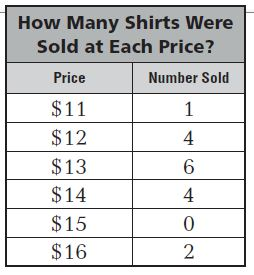Question 1.
How many shirts sold for $12? 4 shirts Answer: 4 shirts Explanation: From the above table, we can say that the no. of shirts sold for$12 is 4

Question 2.
At which price were the most shirts sold?
$________ Answer:$13

Explanation:

The table shows that the most number of shirts sold for $13 Question 3. How many shirts in all were sold? _________ shirts Answer: 17 shirts Explanation: Number of shirts for$11 = 1
Number of shirts for $12 = 4 Number of shirts for$13 = 6
Number of shirts for $14 = 4 Number of shirts for$15 = 0
Number of shirts for $16 = 2 Total no. of shirts sold in all = 1 + 4 + 6 + 4 + 2 = 17 Question 4. How many shirts were sold for$13 or more?
_________ shirts

Explanation:

Number of shirts for $13 = 6 Number of shirts for$14 = 4
Number of shirts for $15 = 0 Number of shirts for$16 =  2
Total no. of shirts sold for $13 or more = 6 + 4 + 2 = 12 shirts Problem Solving Use the line plot above for 5–6. Question 5. Were more shirts sold for less than$13 or more than $13? Explain. ________ Answer: more than$13; 6 > 5

Explanation:

No. of shirts sold for less than $13 = 5 No. of shirts sold for more than$13 = 6
More shirts are sold for more than $13 Question 6. Is there any price for which there are no data? Explain.$ ________

Explanation:

There are no Xs above $15, there were no shirts sold for$15

### Use and Make Line Plots Lesson Check Page No 130

Question 1.
Pedro made a line plot to show the heights of the plants in his garden. How many plants are less than 3 inches tall?
Options:
a. 4
b. 5
c. 10
d. 16

Explanation:

Number of plants of 1 inch = 6
Number of plants of 2 inches = 4
So, the number of plants less than 3 inches tall = 6 + 4
= 10 plants
So, the correct answer is option C

Question 1.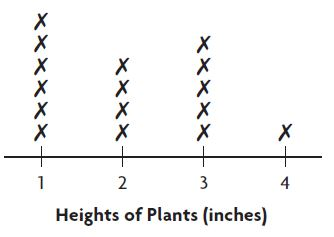Question 2.
Find the sum.
6 4 2
+ 2 5 9
———
Options:
a. 383
b. 801
c. 891
d. 901

Explanation:

Sum of 642 and 259 is 901
Thus the correct answer is option D

Question 3.
Find the difference.
4 6 0
– 3 0 9
———
Options:
a. 61
b. 151
c. 161
d. 169

Explanation:

To get the answer we have to subtract 309 from 460
460 – 309 = 151
Thus the correct answer is option B

Question 4.
There were 262 hamburgers cooked for the school fair. What is 262 rounded to the nearest hundred?
Options:
a. 200
b. 260
c. 270
d. 300

Explanation:

If the digit to the right is more or equal than 5, then the digit in the rounding place increases by one
Change all the digits to the right of the rounding place to zero.
262 rounded to the nearest hundred is 300

Question 5.
Makenzie has 517 stickers in her collection. What is 517 rounded to the nearest ten?
Options:
a. 500
b. 510
c. 520
d. 600

Explanation:

Makenzie has 517 stickers in her collection
If the digit to the right is more or equal than 5, then the digit in the rounding place increases by one
517 rounded to the nearest ten is 520

### Review/Test Page No 131

Question 1.
Mia made a tally table to record the different types of birds she saw at the bird feeder in the garden.For numbers 1a–1c, select True or False for each statement.
a. Mia saw twice as many sparrows as blackbirds.
i. True
ii. False

Explanation:

Use the tally provided in the above table
No. of Sparrows = 12
No. of Blackbird = 6
By this, we can say that the sparrows are twice as blackbirds

Question 1.
b. Mia saw 8 finches.
i. True
ii. False

Explanation:

The above tally table shows that the number of finches = 8

Question 1.
c. Mia saw 4 fewer jays than blackbirds.
i. True
ii. False

Explanation:

No. of Blackbirds = 6
No. of Jays = 4
To know whether the question is true or false
We have to subtract 4 from 6
6 – 4 = 2

Question 2.
Jake asked 25 students in his class how close they live to school. The frequency table shows the results.Part A
Complete the table and explain how you found the answer.

Explanation:

Total Number of students = 25
Now we have to add the number of students from the table
4 + 5 + 4 + 3 + 2 = 18 students
Next, subtract 18 from the total number of students, 25, to find x
25 – 18 = 7
Therefore, the missing number x is 7

Question 2.
Part B
How many more students live about 2 miles or less from school than students who live about 3 miles from school? Show your work.
________ students

Explanation:

Number of students who live about 1 mile = 4 boys + 5 girls = 9 students
Students who live about 2  miles = 4 students
Students who live about 3 miles = 3 boys + 2 girls = 5 students
Next, we have to add total students who live about 2 miles or less = 9 + 4 = 13 students

### Review/Test Page No 132

Use the picture graph for 3–6.

Students at Barnes School are performing in a play. The picture graph shows the number of tickets each class has sold so far.Question 3.
How many tickets were sold altogether? Explain how you found the total.
________ tickets

Explanation:

Number of tickets sold in Ms. Brown’s Class = 9 ✓
Each tick = 5 tickets
5+5+5+5+5+5+5+5+5 = 45 tickets
Number of tickets sold in Mrs. Gold’s Class = 5 ✓
5+5+5+5+5 = 25 tickets
Number of ticks sold in Mr. Castro’s Class = 6 ✓
Each tick = 5 tickets
5+5+5+5+5+5 = 30
Now, we have to add the total number of tickets sold = 45 + 25 + 30 = 100 tickets

Question 4.
Choose the name from each box that makes the sentence true.
Five fewer tickets were sold byclass than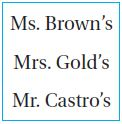class.
Type below:
________

Answer: Mrs. Gold’s Class than Mr. Castro’s Class

Explanation:

Number of tickets sold in Mrs. Gold’s Class = 5 ✓
5+5+5+5+5 = 25 tickets
Number of ticks sold in Mr. Castro’s Class = 6 ✓
Each tick = 5 tickets
5+5+5+5+5+5 = 30
Subtract Number of tickets sold in Mrs. Gold’s from Mr. Castro’s Class
We get 30 – 25 = 5 tickets

Question 5.
How many more tickets were sold by Ms. Brown’s class than Mr. Castro’s class?
_______ tickets

Explanation:

Each tick = 5 tickets
Number of tickets sold in Ms. Brown’s Class = 9 ✓
5+5+5+5+5+5+5+5+5 = 45 tickets
Number of ticks sold in Mr. Castro’s Class = 6 ✓
5+5+5+5+5+5 = 30
Now subtract Number of ticks sold in Mr. Castro’s from Ms. Brown’s Class
45 – 30 = 15 tickets

Question 6.
What if Mrs. Gold’s class sold 20 more tickets? Draw a picture to show how the graph would change.
Type below:
_________

Answer: 20 tickets mean 5 + 5 + 5 + 5, or 4 ✓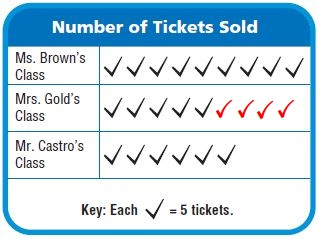So we would add 4 more ticks to Mrs. Gold’s Class

### Review/Test Page No 133

Use the frequency table for 7–8.

Question 7.
The Pet Shop keeps track of the number of fish it has for sale. The frequency table shows how many fish are in three tanks.Part A
Use the data in the table to complete the picture graph.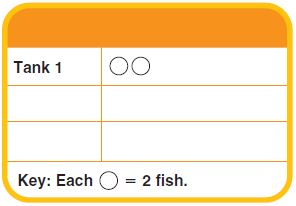Type below:
_________

Given each circle= 2 fishes
Tank 1:
Tank 1 contains 16 fishes
That means 2+2+2+2+2+2+2+2 = 8 circle
Tank 2:
Tank 2 contains 9 fishes
= 2+2+2+2+1 = 4 and a half circle
Tank 3:
Tank 3 contains 12 fishes = 2+2+2+2+2+2 = 6 circle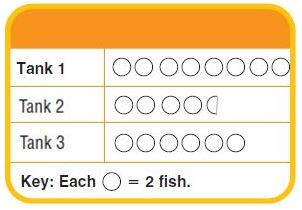Question 7.
Part B
How many pictures did you draw for Tank 2? Explain.
Type below:
________

Answer: 4 and a half circle

Explanation:

Tank 2 contains 9 fishes
Each circulet= 2 fishes
2+2+2+2+1
Therefore the answer is 4 and a half circle

Question 8.
Each tank can hold up to 20 fish. How many more fish can the Pet Shop put in the three tanks?
Options:
a. 60 fish
b. 23 fish
c. 20 fish
d. 33 fish

Explanation:

Given that each tank can hold up to 20 fishes
Total number of tanks = 3
20+20+20 = 60 fishes
From the above table, we observe that
Tank 1 contains 16 fishes
Tank 2 contains 9 fishes
Tank 3 contains 12 fishes
Total number of fishes that all tanks contain = 12+16+9 = 37 fishes
Now, we have to subtract the number of fishes that all tanks contain from the number of fishes pet shop put in the three tanks
= 60 – 37 = 23 fishes

### Review/Test Page No 134

Use the bar graph for 9–12.Question 9.
Three more students play the piano than which other instrument?
__________

Explanation:

The above bar graph shows the number of students who play musical instruments
From the graph, we observe that the number of students who play the flute is 6
And the number of students who play the piano is 9
Subtract Number of students play flute from piano
We get,
9 – 6 = 3

Question 10.
The same number of students play which two instruments?
__________
__________

Explanation:

The graphs the students who play drums and guitar are the same
Because the no. of students who play guitar = 10
And the no. of students who play drums = 10

Question 11.
For numbers 11a–11d, select True or False for each statement.
a. Ten more students play guitar than play flute.
i. True
ii. False

Explanation:

No. of students who play guitar = 10
No. of students who play flute = 6
The statement is not correct

Question 11.
b. Nine students play piano.
i. True
ii. False

Explanation:

The bar graph given in the above shows that the number of students who play piano is 9. So, the answer is true.

Question 11.
c. Six fewer students play flute and piano combined than play drums and guitar combined.
i. True
ii. False

Explanation:

No. of students who play guitar = 10
No. of students who play drums = 10
No. of students who play flute = 6
No. of students who play piano = 9
Now, add the number of students who play flute and piano = 6+9 = 15
Next, add the No. of students who play drums and guitar = 10+10 = 20
The difference between them is 5, not 6

Question 11.
d. Nine more students play piano and guitar combined than play drums.
i. True
ii. False

Explanation:

No. of students who play piano = 9
No. of students who play guitar = 10
Total = 10+9 = 19 students
No. of students who play drums = 10
Subtract No. of students who play drums from total students who play piano and guitar combined
That means 19 – 10 = 9

Question 12.
There are more students who play the trumpet than play the flute, but fewer students than play the guitar. Explain how you would change the bar graph to show the number of students who play the trumpet.
Type below:
________

There are 6 students who play the flute and 10 students who play guitar
The no. of students who play trumpet must be between 6 and 10 i.e., 7, 8, or 9 students.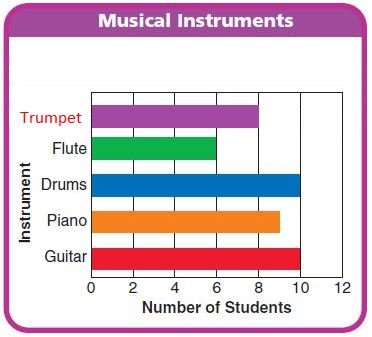In the above example, we show the number of students who play the trumpet is 8

### Review/Test Page No 135

Use the frequency table for 13–14.Question 13.
Part A
Use the data in the table to complete the bar graph.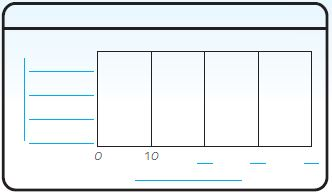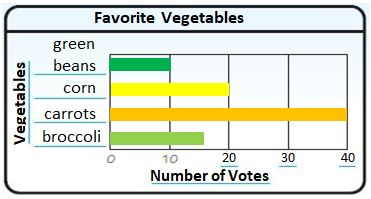Question 13.
Part B
How do you know how long to make the bars on your graph? How did you show 15 votes for broccoli? Explain.
Type below:
_________

By reading Karen’s frequency table we can see that the number of votes for each favorite vegetable.
15 lies between 10 and 20. So, the bar should be drawn all the way to the midpoint between 10 and 20.

Question 14.
How many more votes did the two most popular vegetables get than the two least popular vegetables? Explain how you solved the problem.

Explanation:

The two most popular vegetables are carrots and corn
And the number of votes for carrots and corn are 40 and 20
The two least popular vegetables are broccoli and green beans
And the number of votes for broccoli and green beans are 15 and 10
Now, Subtract the number of votes for broccoli and green beans from a number of votes for carrots and corn
60-25 = 35 students

### Review/Test Page No 136

Use the line plot for 15–16.

The line plot shows the number of goals the players on Scot’s team scored.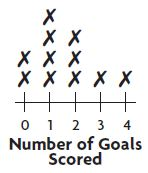Question 15.
For numbers 15a–15d, select True or False for each statement.
a. Three players scored 2 goals.
i. True
ii. False

Explanation:

One player scored 2 goals, one player scored 4 goals and one player scored 3 goals

Question 15.
b. Six players scored fewer than 2 goals.
i. True
ii. False

Explanation:

From the figure, we can say that 4 players scored 1 goal and 2 players scored 0
4+2 =6

Question 15 (request help)
c. There are 8 players on the team.
i. True
ii. False

Explanation:

We need to count all X = 11

Question 15
d. Five players scored more than 1 goal.
i. True
ii. False

Explanation:

More than 1 goal means 2, 3 or 4 goals
We observe that 3 players who scored 2 goals, 1 player who scored 3 goals, 1 player who scored 4 goals
Now we have to add the players who scored more than 1 goal
3+1+1 = 5
Therefore 5 players scored more than 1 goal

Question 16.
What if two more people played and each scored 3 goals? Describe what the line plot would look like.
Type below:
__________

Answer: We have to add two more X on the line plot 3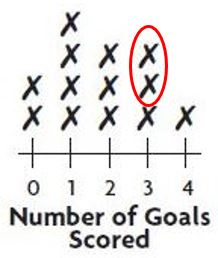Use the line plot for 17–18.

Robin collected shells during her vacation. She measured the length of each shell to the nearest inch and recorded the data in a line plot.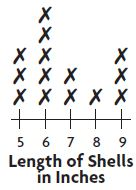Question 17.
How many shells were 6 inches long or longer?
_________ shells

Explanation:

5 shells were 6 inches long
2 shells were 7 inches long
1 shell was 8 inches long
3 shells were 9 inches long
Total = 5+2+1+3 = shells
Thus the answer is 11 shells

Question 18.
How many more shells did Robin collect that were 5 inches long than 8 inches long?
________ shells